Next: Hidden Markov Random Field Up: Hidden Markov Random Field Previous: Finite Mixture Model

## Markov Random Field Theory

The spatial property can be modelled through different aspects, among which, the contextual constraint is a general and powerful one. Markov random field (MRF) theory provides a convenient and consistent way to model context-dependent entities such as image pixels and correlated features. This is achieved by characterizing mutual influences among such entities using conditional MRF distributions. In an MRF, the sites in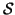are related to one another via a neighbourhood system, which is defined as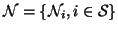, where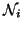is the set of sites neighbouring i,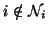and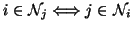. A random field X said to be an MRF onwith respect to a neighbourhood system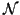if and only if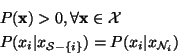Note, the neighbourhood system can be multi-dimensional. According to the Hammersley-Clifford theorem , an MRF can equivalently be characterized by a Gibbs distribution. Thus,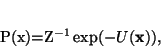(5)

where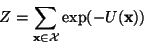(6)

is a normalizing constant called the partition function, and U(x) is an energy function of the form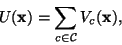(7)

which is a sum of clique potentials Vc(x) over all possible cliques. A clique c is defined as a subset of sites inin which every pair of distinct sites are neighbours, except for single-site cliques. The value of Vc(x) depends on the local configuration on clique c. For more detail on MRF and Gibbs distribution see .Next: Hidden Markov Random Field Up: Hidden Markov Random Field Previous: Finite Mixture Model
Yongyue Zhang
2000-05-11